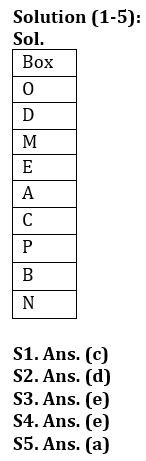Latest Banking jobs   »

# Reasoning Ability Quiz For LIC ADO Prelims 2023- 8th March

Directions (1-5): Study the information carefully and answer the questions given below.
Nine boxes are placed in a stack one above another. Not more than three boxes placed below box C. Three boxes are placed between box C and box D. Box O is placed just above box D. Box P is placed above box B and below box E. Box N is placed at bottom. Box A is placed above box C and below box E. More than four boxes are placed between box N and box M. Neither box A nor box M is placed at top of stack. Not more than one box is placed between box M and box A.

Q1. Which of the following box is placed just above box C?
(a) Box B
(b) Box P
(c) Box A
(d) Box N
(e) None of these

Q2. Which of the following box is placed just below box P?
(a) Box N
(b) Box C
(c) Box M
(d) Box B
(e) None of these

Q3. How many boxes are placed in between box D and Box B?
(a) One
(b) Four
(c) Two
(d) Three
(e) More than Four

Q4. Four of the following five are alike in certain way based from a group, find the one that does not belong to that group?
(a) E-C
(b) P-N
(c) M-A
(d) O-M
(e) D-P

Q5. Which of the following box is placed at top of stack?
(a) Box O
(b) Box D
(c) Box M
(d) Box P
(e) None of these

Directions (6-10): Study the following arrangement carefully and answer the questions given below:

2 3 5 7 1 2 6 4 5 7 1 9 8 5 2 4 1 4 5 8 2 6 3 7 6 9 4 1 5 6 7

Q6. Which of the following digit will be seventh to the right of eleventh from the left end?
(a) 2
(b) 4
(c) 5
(d) 7
(e) None of these

Q7. How many 2’s are there in the series which are followed by a number, which is less than or equal to 5?
(a) One
(b) None
(c) three
(d) Two
(e) More than three

Q8. What will be the addition of fourth and twelfth digit from the right end and eleventh digit from the left end?
(a) 12
(b) 11
(c) 13
(d) 10
(e) None of these

Q9. If all the 2’s and 4’s are removed from the series, then which of the following will be fifth to the right of twelfth digit from the left end?
(a) 7
(b) 3
(c) 8
(d) 6
(e) None of these

Q10. How many 7’s are there in the series which are immediately preceded by an odd number and immediately followed by a number?
(a) One
(b) None
(c) three
(d) Two
(e) More than three

Directions (11-15): The following questions are based on the five three – digit numbers given below:

924 574 456 187 675

Q11. If 3 is added to the second digit of each of the numbers how many numbers thus formed will be divisible by three?
(a) None
(b) One
(c) Two
(d) Three
(e) None of these

Q12. If all the digits in each of the numbers are arranged in descending order within the number, which of the following will be the highest number in the new arrangement of numbers?
(a) 924
(b) 574
(c) 456
(d) 187
(e) 675

Q13. What will be the resultant number if the third digit of the second highest number is divided by the 2nd digit of the second lowest number?
(a) 2
(b) 3
(c) 0
(d) 1
(e) 4

Q14. If 3 is added to the first digit and 1 is added to the last digit of each of the number then which of the following numbers will be the second highest number?
(a) 924
(b) 574
(c) 456
(d) 187
(e) 675

Q15. If in each number the first and the third digits are interchanged then which will be the highest number?
(a) 924
(b) 574
(c) 456
(d) 187
(e) 675

SolutionsSolutions (6-10):
S6. Ans. (b)
Sol.
The element seventh to the right of eleventh from the left end is (11+7) = 18th from the left end i.e., is ‘4’.

S7. Ans. (d)
Sol. There are only two 2’s in the series which are followed by a number, which is less than or equal to 5 i.e., ‘2 3’ and ‘2 4’.

S8. Ans. (d)
Sol.
The addition of fourth and twelfth element from the right = (1+8) = 9.
The eleventh digit from the left end is = 1
Hence, addition is = (9+1) = 10

S9. Ans. (a)
Sol.
After removing 2’s and 4’s is
3 5 7 1 6 5 7 1 9 8 5 1 5 8 6 3 7 6 9 1 5 6 7
Then, the element fifth to the right of twelfth from the left end is ‘7’.

S10. Ans. (c)
Sol.
There are only three 7’s which are immediately by an odd number and immediately followed by a number i.e., ‘5 7 1’, ‘5 7 1’ and ‘3 7 6’.

Solutions (11-15):
S11. Ans. (d)
S12. Ans. (a)
S13. Ans. (d)
S14. Ans. (e)
S15. Ans. (d)## FAQs

### When will the LIC AAO Prelims Exam 2023 be conducted?

The LIC AAO Prelims Exam 2023 will be conducted on 17th & 20th February 2023.

#### Congratulations!Union Budget 2023-24: Free PDF